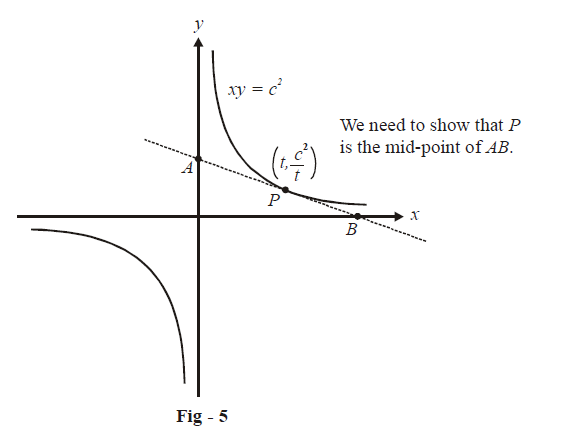# Examples on Tangents and Normals Set 1

Go back to  'Applications of Derivatives'

### Example - 1

Prove that the segment of the tangent to $$xy={{c}^{2}}$$  intercepted between the axes is bisected at the point of contact.

Solution: Let us take an arbitrary point on this curve, \begin{align}\left( {t,\frac{{{c^2}}}{t}} \right)\end{align}. An approximate figure showing the tangent at this point is sketched below:The procedure that we need to follow is first determine the equation of the tangent at the point P, find the intercepts this tangent makes with the axes (we will then get the co-ordinates of the points A and B), and show that P is the mid-point of AB.

\begin{align}{m_T}\left( {{\rm{at}}\,P} \right) = {\left. {\frac{{dy}}{{dx}}} \right|_{x = t}} = {\left. {\frac{{ - {c^2}}}{{{x^2}}}} \right|_{x = t}} = \frac{{ - {c^2}}}{{{t^2}}}\end{align}

\begin{align} & \mathbf{Equation}\, \mathbf{of}\text{ }\mathbf{tangent}: \qquad \qquad \qquad y-\frac{{{c}^{2}}}{t}=\frac{-{{c}^{2}}}{{{t}^{2}}}\left( x-t \right) \\\\ & \mathbf{Point}\text{ }\mathbf{A}:\qquad\qquad\text{Put}\qquad \qquad \qquad x=\text{ }0 \\\\ &\qquad \qquad \qquad \qquad \quad \Rightarrow \qquad \qquad \qquad \text{ }\!\!~\!\!\text{ }y=\frac{{{c}^{2}}}{t}+\frac{{{c}^{2}}}{t}=\frac{2{{c}^{2}}}{t} \\\\ & \qquad \qquad \qquad \qquad \quad \Rightarrow \qquad \qquad \qquad\text{The point A is}\left( 0,\frac{2{{c}^{2}}}{t} \right) \\\\ & \mathbf{Point}\text{ }\mathbf{B}: \qquad \qquad \text{Put} \qquad \qquad \qquad y=\text{ }0 \\\\ & \qquad \qquad \qquad \qquad \quad\Rightarrow \qquad \qquad \text{ }~\text{ }~\text{ }~\text{ }x=t+t=2t \\\\ & \qquad \qquad \qquad \qquad \quad \Rightarrow \qquad \qquad \text{ }~\text{ }~\text{ }~\text{ The point B is }(2t,0)\end{align}

\begin{align} & \mathbf{Mid}-\mathbf{point}\text{ }\mathbf{of}\;AB:~\text{ The mid-point of}\,AB\,\text{is} \\\\ & \qquad \qquad \qquad \qquad \qquad \qquad \left( \frac{0+2t}{2},\frac{\begin{align}\frac{2{{c}^{2}}}{t}\end{align}+0}{2} \right) \\\\ & \qquad \qquad \qquad \qquad \qquad \qquad =\left( t,\frac{{{c}^{2}}}{t} \right) \\\\ & \qquad \qquad \qquad \qquad \qquad \text{which is the same as the point}\,P. \\\\ &\qquad \qquad \qquad \qquad \qquad \Rightarrow \quad \text{P }\text{ is the mid-point of }~\text{ }AB \\ \end{align}

### Example - 2

Find the equations of tangents to the curve $$y = x^4$$  which are drawn from the point (2, 0).

Solution: We write the equation of the tangent to  $$y =x^4$$  at a general point $$(t,~{{t}^{4}})$$ and then make (2, 0) satisfy that equation.

\begin{align}{m_T} = {\left. {\frac{{dy}}{{dx}}} \right|_{x = t}} = {\left. {4{x^3}} \right|_{x = t}} = 4{t^3}\end{align}

\begin{align}&\mathbf{Equation}\text{ }\mathbf{of}\text{ }\mathbf{tangent}:\qquad \qquad y-{{t}^{4}}=4{{t}^{3}}\left( x-t \right) \\\\& \qquad \qquad \qquad \qquad\qquad\quad \Rightarrow \qquad 4{{t}^{3}}x-y-3{{t}^{4}}=0\qquad\qquad\qquad...(\text{i}) \end{align}

Since the tangent we require passes from (2, 0), the co-ordinates of (2, 0) must satisfy (i)

\begin{align}&\Rightarrow \qquad 4{t^3}\left( 2 \right) - \left( 0 \right) - 3{t^4} = 0\\&\Rightarrow \qquad 8{t^3} - 3{t^4} = 0\\&\Rightarrow \qquad {t^3}\left( {8 - 3t} \right) = 0\\&\Rightarrow \qquad t = 0,\frac{8}{3} \end{align}

From(i), the two possible tangents are (corresponding to the two values of t):

\begin{align}y = 0;\,\,\,\,\,\,y - {\left( {\frac{8}{3}} \right)^4} = 4{\left( {\frac{8}{3}} \right)^3}\left( {x - \frac{8}{3}} \right) \end{align}

### Example – 3

Find the point(s) on the curve $${y^3} + 3{x^2} = 12y$$  where the tangent is vertical.

Solution: A vertical tangent means that the slope of the tangent is $$\infty$$.

Differentiating the equation of the given curve w.r.t x, we get:

\begin{align}&3{y^2}\frac{{dy}}{{dx}} + 6x = 12\frac{{dy}}{{dx}}\\\\\Rightarrow \qquad &\frac{{dy}}{{dx}} = \frac{{6x}}{{12 - 3{y^2}}}\end{align}

Hence, for vertical tangent:

\begin{align}&\qquad \qquad \qquad 3{y^2} = 12\\\\&\Rightarrow \qquad \qquad \;\;y = \pm \,2\\\\&\Rightarrow \qquad \qquad \;3{x^2} = y\left( {12 - {y^2}} \right)\\\\&\qquad \qquad \qquad \quad \; = \pm \,\,16\\\\&\Rightarrow \qquad \quad \quad \;\; x=\pm \frac{4}{\sqrt{3}}\left( \text{for}\,\,y=2 \right)\;\quad\text{ }\!\!\{\!\!\text{ For y = }-2,\text{x has imaginary values}\}\end{align}

Therefore, the required points are \begin{align}\left( { \pm \frac{4}{{\sqrt 3 }},2} \right)\end{align}